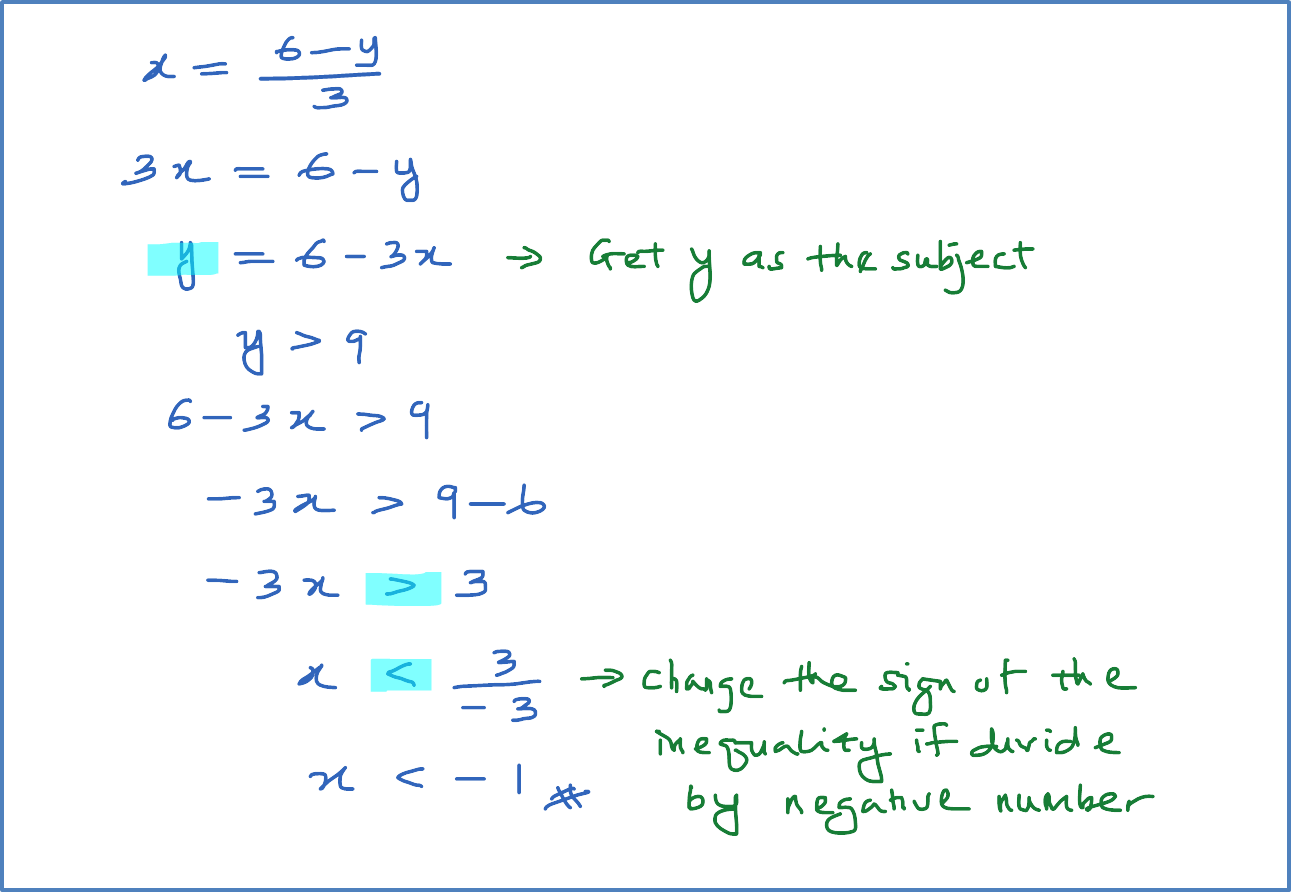# 3.6.2 Linear Inequality – Example 1

(C) Linear Inequality

Example 1
(a)  Given $x=\frac{6-y}{3}$  , find the range of values of x for which y > 9 .
(b) Given $2x+3y-6=0$  , find the range of values of x for which y < 4 .